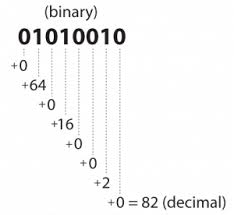Problems
301 - Binary to Decimal

Binary to Decimal

Time Limit: 1 sec

The Problem

In mathematics and digital electronics, a binary number is a number expressed in the binary numeral system, or base-2 numeral system, which represents numeric values using two different symbols: typically 0 (zero) and 1 (one).

Write a simple program which read a 5 digit binary number X and convert the binary number to decimal then print the decimal number.

Example:

if X = 10111

Calculation is very simple

1 X 24 +  0 X 23 + 1 X 22 + 1 X 21 + 1 X 20

= 1 X 16 + 0 X 8 + 1 X 4 + 1 X 2 + 1 X 1

= 16 + 0 + 4 + 2 + 1

= 23The Input

The input file contains only one 5 digit binary number.

The Output

Output will show the one integer number wich is the converted decimal value from the given binary in a separate line.

Sample Input

10111

Sample Output

23

Problem Setter: Shahin ShamS